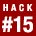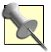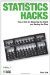# Hack 15. Identify Unexpected OutcomesHow do you know if your observations are correct or if you are just biased? How do you know when there is more or less of something than should have occurred by chance? You can find out for sure by using the flexible one-way chi-square test.

In science, the oldest type of observational research involved counting people, animals, and things:

• How many people are on this boat?

• What proportions of butterflies have little green spots on their wings?

As the field of inferential statistics matured, the questions became more specific:

• Were an equal number of boys and girls born in London in 1812?

• Are an equal number of crimes committed at different times of day?

The research question for these situations is "are they equal?" (or, at least, are they close enough that any fluctuations are probably due to chance). The implication of an unequal distribution is that something is going on. What, exactly, is going on cannot be answered by this sort of question. It is a start, though, and a good first question to ask.

Have you ever noticed that something seemed to be going on, but weren't sure if it was just your imagination? Do a greater number of hippies shop at the local community mercantile than would be expected by chance? If the answer is yes, and you are looking to meet hippies, you should start hanging out there.

In business, and for those who have to provide services, identifying where there is the most need is crucial. Observational data can be used to solve that problem. Even just in everyday life, we all have our beliefs (which might be biased) that are based on observations. I have noticed a lot of hippies at the community mercantile, but maybe I am just on the lookout for hippies when I am in that store. Are there really more hippies than normal there? More hippies than, say, nonhippies?

These sorts of questions can be answered using a statistical tool appropriate for seeing whether the number of "things" within each of a number of categories is more unequal than would normally be found by chance. This tool is named the one-way chi-square.This statistical analysis is called chi-square because the symbol used for the critical value generated is an C, which is the Greek letter chi (pronounced "kye"). The values needed in the calculations are all squared, thus we call this whole thing a chi-square or chi-squared.

#### Determining Whether Something Is Going On

Imagine you are responsible for scheduling the police officers in your town. The problem is that you don't know whether to schedule the same amount of officers for every shift or whether more crime might occur during particular shifts. If one shift is likely to be busier, you should probably assign more officers. Of course, another reason to assign more officers during that time is that their patrolling might cut crime down a bit.

Here is an example of some imaginary data describing crime events for three periods of time. Imagine the data was collected over a 30-day period, and you would like to use this data to plan for the coming year. The numbers indicate how many crimes were committed during each of three police shifts.

Midnight - 8 a.m.8 a.m. - 4 p.m.4 p.m. - MidnightTotal
1209090300

It certainly looks like more crimes occur late at night. By observation alone, we might conclude that there is more crime late at night. Perhaps that is just in our sample, though, and there really isn't a difference in the population of all the data we could have collected.

#### Calculating the Chi-Square

We could compute a chi-square for this data. If the chi-square is really big, then the 120 crimes is unusually larger than the other two crime periods. How big "really big" needs to be is an important question that we will explore later in this hack.Here's how to think about the analysis we are about to do. If there are 300 crimes committed in one 24-hour period, we would expect 33.3 percent of them, or 100, to occur in each of three equally long time intervals during the day. If there is more or less than 100 for each of those intervals, something is going on. Perhaps the time of day matters in the commission of crimes. Of course, there might be some chance fluctuation, but the larger the difference between the expected and the actual frequencies, the less likely that those differences are just chance.

Here is the chi-square formula:

S is a symbol that means to sum or add up the things that follow it.

Let's calculate a chi-square for this data. The observed frequency for each category is given. The expected frequency for each cell would be 300 divided by three categories, or 100:

The chi-square for this data is 6. Okay. Now what? Is 6 big or small or what? Could a chi-square as big as 6 occur by chance?

#### Determining if the Chi-Square Is "Really Big"

As with all statisticssuch as correlation coefficients [Hack #11], t tests [Hack #17], proportions, and so onstatisticians have mapped out the distribution of the chi-square. In other words, we know the likelihood that chi-squares of different sizes will occur by chance. The likelihood of finding chi-squares of particular magnitudes depends on the number of categories.

Table 2-5 shows a portion of a theoretically giant table that shows the chi-square values that one must beat in order to be 95 percent sure (level of significance = .05) that the value didn't get that big just because of chance fluctuations in the sample. We know these critical values occur by chance 5 percent or less of the time because chi-squares, like almost everything else in the orderly world of statistics, have a known distributioni.e., a known set of likelihoods that certain values will occur. Like the normal curve, the chi-square distribution is well-defined [Hack #23].

##### Table Critical chi-square values at the .05 level of significance
Two categoriesThree categoriesFour categoriesFive categories
3.845.997.829.49

Our chi-square value is 6, which is higher than the critical value for three categories (5.99). This means something very specific, so I'll emphasize it. Though I am specifically referring to the crime rate problem at hand, I am using the same pattern of words that describe all statistical findings that are significant at the .05 level.If, in the population, there are no differences in the number of crimes committed at the three times of day, you would occasionally draw out random samples with differences that produce a chi-square of 6 or larger, but it would happen less than 5 percent of the time.

It seems reasonable to conclude, then, that in the population there are differences in frequency of crime based on time of day. Because these differences are "real," it is reasonable to schedule a year's worth of police patrols based on them.

#### Why It Works

Data for chi-square analyses are laid out in a way in which the observed number of things in each category can be compared with the expected number of things in each category. The "expected number of things in each category" is usually defined as an equal number. If nothing is going on (i.e., if the category makes no difference), we expect an equal number of things in each category.

Chi-squares work with categorical data. Essentially, the difference between what was expected and what was observed is computed for each category. The differences are compared to the expected frequency (as a way to standardized all the differences), and then those ratios are all added together. The size of the resulting number determines its likelihood of occurring by chance. The bigger the number, the less likely that chance alone explains things. There is a known distribution (list of probabilities associated with each possible chi-square value) that is used by a table (or computer) to assign a specific probability to each chi-square value.

If there are two or more categories and the researcher wants to know whether the actual distribution across these categories is what would be expected by chance alone, then the chi-square is an appropriate test. The actual value that is tested is the difference between what the researcher expects to find and what actually occurs.

The chi-square test is used in the framework of having certain expectations and seeing whether they are met by the observed data. This is a simple form of model testing. The researcher has a belief system, in the form of some model or hypothesis of how the world should behave. She then observes the world (collects data) and compares her observations to her model. If the data fits the model, this is support for her hypotheses. The chi-square test, consequently, is considered a goodness-of-fit statistic. It answers the question of how well the data fits a model.Some statistics textbooks refer to the one-way chi-square as the single sample chi-square, so don't get confused. But what are you doing reading some other statistics book anyway?

Statisticians know the size of normal fluctuations in observed frequencies compared to expected frequencies. With this knowledge, they can compute the likelihood that any observed deviation from the expected occurs by chance or because something else is going on.

#### Where Else It Works

Though a simple and historically ancient (about 80 years old, which is old by statistics standards!) statistical method, the chi-square is very useful for a variety of statistical questions at both low levels of measurement and, surprisingly, very advanced statistical methods. Because it is a fairly straightforward way to model test (or quantify "goodness of fit"), the chi-square is used as part of complex correlational analyses and measurement diagnostics.

Chi-square analyses are used to see whether complicated theoretical models of the worldcomprehensive maps of relationships among variablesactually match real-world data. If the real world deviates too much from the expectations implied by one of these models, it is concluded that the model is weak. A significant chi-square is the criterion used for "too much" deviation.

For example, if test developers are concerned about item bias (that one item might work differently for one identifiable group over anothersuch as races, genders, and so on), they will check whether the patterns of answer options meet certain expectations regardless of which group generated the data. The chi-square analysis compares the expectations to actual test performance.

• "Identify Unexpected Relationships" [Hack #16]Statistics Hacks: Tips & Tools for Measuring the World and Beating the Odds
ISBN: 0596101643
EAN: 2147483647
Year: 2004
Pages: 114
Authors: Bruce Frey

Similar book on Amazon